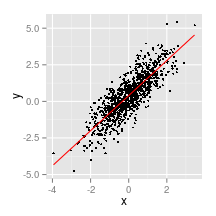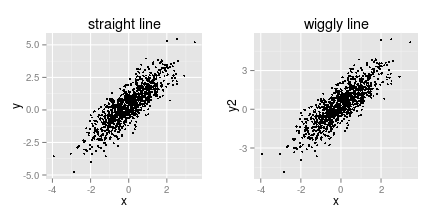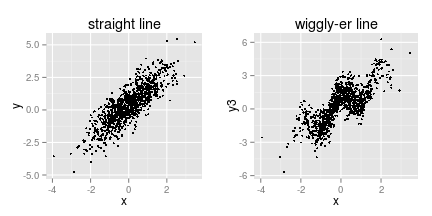On Regression: Part I

# On Regression: Part I

Everyone loves doing regressions, but what are they? In the most general sense, a regression model says that the response $$y$$ is a noisy observation of a function $$f: \mathbb{R}^p \to \mathbb{R}$$ whose argument is the vector $$x$$. So if we have $$n$$ observations of $$y$$ and the $$p$$-vector $$x$$, then the $$i^{th}$$ entry of $$y$$ can be written

$y_i = f(x_i) + \epsilon_i,$

with $$\epsilon_i \sim G$$. $$G$$ is just some probability law and $$f$$ is some function, I've introduced no other conditions. $$G$$ might not have a density and $$f$$ may not be continuous. In practice, one usually assumes that the $$\epsilon$$'s are $$iid$$ from $$G$$, but they need not be, as in time series models, for example. For now, we'll stick with $$iid$$ errors, a pretty mild assumption unless we're working with spatial or longitudinal data or something like that. Really, I just want to impress that if I'm interested in understanding how variable $$y$$ depends on variables $$x_1,\ldots,x_p$$, using a regression model can be very flexible and allow for most of the behavior that one could reasonably expect, depending on how we model $$f$$ and $$\epsilon$$.

That's a much more general setting than that in which most of us think of regression, and for the first couple of years I spent becoming a data nerd, I didn't mean that either when I talked about regression, I just meant a linear model. So let's start with the specific and familiar and work our way toward the general and the weird. Our old friend the Gaussian linear model is just

$y_i = x_i \beta + \epsilon_i$

with $$\epsilon_i \sim N(0,\sigma^2)$$. This is the simplest regression model, and it's what a lot of software packages refer to as “regression,'' so no wonder the term has been abused a bit. Notice though that we've made two important assumptions: (1) the function $$f$$ is a linear map and (2) the noise is $$iid$$ Gaussian. These assumptions are much stronger: we've picked a specific distribution for $$\epsilon$$ and we've decided that among the myriad functions that exist, ours is going to be linear. This is great if it's a good approximation of the actual relationship between $$x$$ and $$y$$, and may be really terrible if it isn't. Let's have a look at some data generated from this model (so they fit the model exactly).

n <- 1000
x <- rnorm(n)
alpha <- 0.4
bet <- 1.2
y <- alpha + x * bet + rnorm(n, 0, 0.81)
dat <- data.frame(cbind(y, x))
names(dat) <- c("y", "x")
fit <- lm(y ~ x, data = dat)
dat$yhat <- fit$fitted.values

ggplot(dat, aes(y = y, x = x)) + geom_point(size = 1) + geom_line(aes(y = yhat),
colour = 2)The data certainly seem to lie on a line, but it's a little bit of a messy line, and that's because the magnitude of the noise scale (standard deviation of 0.81) is substantial relative to the magnitude of the effect (1.4). What if I make the line wiggly?

dat$y2 <- dat$y + 0.1 * cos(pi * x)
p1 <- ggplot(dat, aes(x = x, y = y)) + geom_point(size = 1) + ggtitle("straight line")
p2 <- ggplot(dat, aes(x = x, y = y2)) + geom_point(size = 1) + ggtitle("wiggly line")

multiplot(p1, p2, cols = 2)Can you tell the difference? No? Good, neither can I. But why not? The line's not straight anymore, I've added a periodic component. We know the model is wrong. The reason is that the magnitude of the periodic component is small relative to the error variance, so it's just lost in the noise. The linear model is a good approximation of what's actually going on in the data. This is a nice, very simple illustration of why I find the very common objection "you're crazy if you think the data actually fit the assumptions of your model” to be funny. The question is not whether the data were generated by the model. It is, after all, just a model, and there's a reason we use the word model instead of “candidate true state of the world.” Rather, the question is whether the model is reasonably close to what's going on in the data. One of the most famous lines in statistics (a fine distinction, if I do say so myself) is George Box's famous quip “all models are wrong, but some are useful.” That is, I think, the only reasonable way to think about it.

Now sometimes we can see very clearly that the model is wrong. Let's just make that periodic component a bit bigger.

dat$y3 <- dat$y + cos(pi * x)
p1 <- ggplot(dat, aes(x = x, y = y)) + geom_point(size = 1) + ggtitle("straight line")
p2 <- ggplot(dat, aes(x = x, y = y3)) + geom_point(size = 1) + ggtitle("wiggly-er line")

multiplot(p1, p2, cols = 2)In upcoming posts I'll go beyond linear models and describe ways to model (1) more general regression functions, like the periodic one above; and (2) non-Gaussian error distributions.

This entry was posted in Uncategorized. Bookmark the permalink.